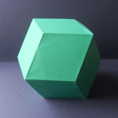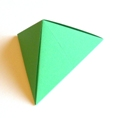Origami Heaven

A paperfolding paradise

The website of writer and paperfolding designer David Mitchell

x

Rhombic Polyhedra

When I was writing my first book, Mathematical Origami, I found that I needed names for several polyhedra, all easily made from simple modules derived from the silver rectangle, for which there did not appear to be an established mathematical name. The obvious characteristics of these polyhedra were that their faces were either 109.28/70.32 rhombuses or 70.32/54.84/54.84 (1:sqrt3/2:sqrt3/2) triangles, which can be obtained by folding the 109.28/70.32 rhombus in half lengthways, or a mixture of the two. For want of better, I stole the word 'rhombic' from the name of the rhombic dodecahedron, whose faces are, of course, 109.28/70.32 rhombuses, and applied it to the other polyhedra as well, thus creating the terms rhombic tetrahedron, rhombic octahedron etc and the general term rhombic polyhedra to describe them as a group. I justified this usage to myself on the grounds that the triangular faces occurred in pairs, which if flattened would come back to a 109.28/70.32 rhombus. Somewhat to my surprise, my publisher raised no objection to this.

I was expecting that I would receive a barrage of criticism for this usage and that someone would soon point out to me a better terminology to use for these forms, but, somewhat strangely, neither of these things have happened. I still, however, expect a better terminology to emerge at some stage.

Pro tem then I shall continue to use the term rhombic polyhedra, although in a slightly developed sense, to mean the set of those polyhedra which can be combined with other identical polyhedra to build a rhombic dodecahedron and which have at least some faces that are either 109.28/70.32 rhombuses or 70.32/54.84/54.84 (1:sqrt3/2:sqrt3/2) triangles. The members of this interesting set of polyhedra are illustrated below. All of them will fill space.

This definition deliberately excludes many polyhedra whose faces are 70.32/54.84/54.84 (1:sqrt3/2:sqrt3/2) triangles, and particularly those that can be made by putting together three-sided pyramids, or sunken pyramids, whose faces are such triangles and similar designs whose faces are or include 109.28/35.36/35.36 (sqrt2:sqrt3/2:sqrt3/2) triangles, which can be obtained from the 109.28/70.32 rhombus by folding it in half in the other direction. If these forms were to be included within the definition of rhombic polyhedra then we would be in a situation where there were, for instance, three separate forms that could quite correctly be called rhombic hexahedra and three more that could quite correctly be called rhombic dodecahedra. This is clearly nonsensical and so I have adopted the narrower definition given above. I have not invented any general name for the excluded forms.

It is worth pointing out that although the 109.28/70.32 rhombus has diagonals in the proportion 1:sqrt2 I have avoided using the term silver rhombus since that would suggest that this rhombus has properties similar to the silver rectangle and silver triangle, which it does not.

The classic book Mathematical Models, by H M Cundy and A P Rollett contains a net for the rhombic pyramid, although the authors do not give it a name. They do, however, use the term rhombic polyhedra in a different sense from mine as a general term for polyhedra whose faces are rhombuses, ie the cube, the rhombic dodecahedron and the triacontahedron. I apologise for introducing confusion by using this term in another way.

The third edition of this book, published in 1960, also contains the information that a Mr Dorman Luke, otherwise unknown to me, has found that all three stellations of the rhombic dodecahedron can be arrived at by adding what I call rhombic pyramids to the rhombic dodecahedron in successively larger numbers. These forms fall outside my definition of rhombic polyhedra but this is not a difficulty as they already have their own established names. I have, incidentally, found that by continuing the process of adding rhombic pyramids to the third stellation it is eventually possible to arrive at a much larger rhombic dodecahedron than the one used to initiate the process.

The remainder of this page provides brief details of those rhombic polyhedra that can be used to build rhombic dodecahedra and the rhombic dodecahedron itself, and describes some of their interesting properties. A selection of forms that can be arrived at by combining rhombic dodecahedra will be added when time allows. Details of modular origami versions of many of these polyhedra and modular sculptures based upon them can be found elsewhere on this site in the Designs by Polyhedral Form section of the Modular Origami Design Encyclopaedia.The Rhombic Dodecahedron has 12 faces, all of which are 109.28/70.32 rhombuses.The volume of a rhombic dodecahedron is twice the volume of a cube inscribed inside it. A rhombic dodecahedron can be built from 4 rhombic hexahedra, 6 rhombic octahedra, 12 rhombic pyramids, 24 rhombic tetrahedra or square base rhombic pyramids or 48 .............The Rhombic Hexahedron has 6 faces, all of which are 109.28/70.32 rhombuses.4 rhombic hexahedra will fit together to form a rhombic dodecahedron. The rhombic hexahedron is a replihedron. A large rhombic hexahedron can be built by combining 8 small ones. A rhombic hexahedron can be built from 3 Rhombic Pyramids.The Rhombic Octahedron has 8 faces, all of which are 70.32/54.84/54.84 triangles.6 rhombic octahedra will fit together to form a rhombic dodecahedron. A rhombic octahedron can be built from 2 rhombic pyramids or square base rhombic pyramids or 4 rhombic tetrahedra.The (Rhombus Base) Rhombic Pyramid has 5 faces, one of which is a 109.28/70.32 rhombus and 4 of which are 70.32/54.84/54.84 triangles.12 rhombic pyramids will fit together to form a rhombic dodecahedron. A rhombic pyramid can be built from 2 rhombic tetrahedra. The rhombic pyramid is a replihedron. A large rhombic pyramid can be built by combining 8 small ones in 3 different ways. 4 rhombic pyramids will go together to form a large rhombic tetrahedron. Rhombic pyramids can also be added to the rhombic dodecahedron to produce its three stellated forms.The Square Base Rhombic Pyramid has 5 faces, one of which is a square and 4 of which are 70.32/54.84/54.84 triangles.12 square base rhombic pyramids will fit together to form a rhombic dodecahedron. 6 square base rhombic pyramids will fit together to make a cube.The Rhombic Tetrahedron has 4 faces, all of which are 70.32/54.84/54.84 triangles.24 rhombic tetrahedra will fit together to form a rhombic dodecahedron. The rhombic tetrahedron is a replihedron. A large rhombic tetrahedron can be built by combining 8 small ones.The Lesser Rhombic Tetrahedron has 4 faces, one of which is a 70.32/54.84/54.84 triangle, 2 of which are 90/54.84/35.16 triangles and the other a 90/45/45 or silver triangle.48 lesser rhombic tetrahedra will fit together to form a rhombic dodecahedron. To borrow a term from another branch of mathematics, the lesser rhombic tetrahedron can be seen as the common denominator of all the other rhombic polyhedra. 2 will go together to form a rhombic tetrahedron and 4 to form a square base rhombic pyramid.